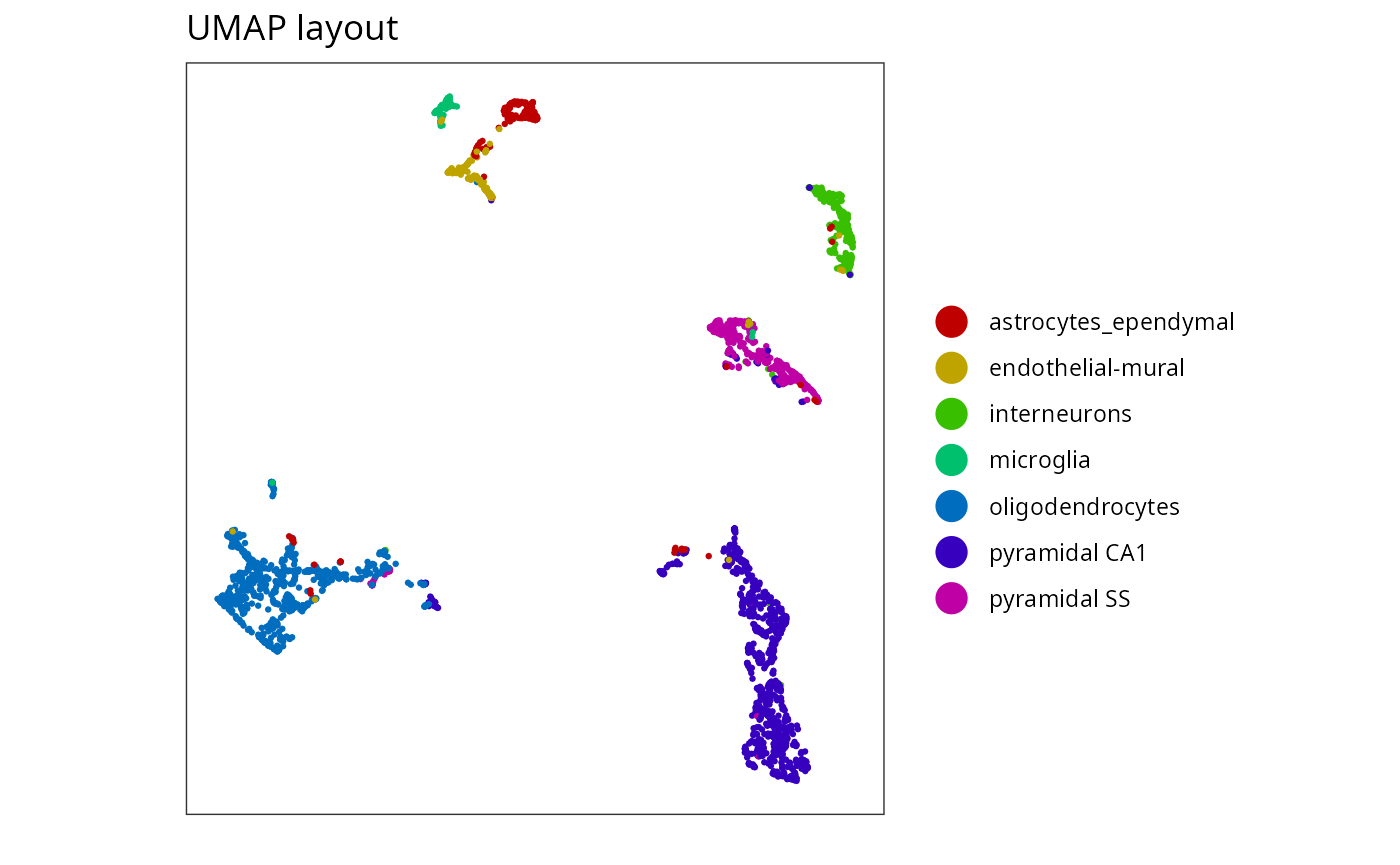library(langevitour)
Single-cell RNA-Seq gene expression of 2,816 mouse brain cells (Zeisel, 2015). The top 10 principal components were produced using the steps in the Bioconductor OSCA workflow. This is unscaled PCA of log$$_2$$(normalized_count+1) expression levels, mean UMI count 15,550 per cell. So $$x$$ units of distance can be explained for example by $$n$$ genes with $$x/\sqrt{n}$$ log$$_2$$ fold change each.
data(zeiselPC)
zeiselPC[,-1], zeiselPC$type, levelColors=palette, elementId="brain1") • Try setting the guide to . • Examine particular cell types in detail by the other cell types. • Get a 3D effect by . ## Mouse brain cells denoised The single cell data is noisy. Typically this sort of data would be examined using a UMAP or t-SNE layout. The UMAP layout below looks very clean. Can we achieve something similar?My current suggestion to reduce noise is, for each point, to take the average of cells reachable in a certain number of steps along the directed k-nearest neighbor graph. See the function knnDenoise(). zeiselDenoised <- knnDenoise(zeiselPC[,-1], k=30, steps=2) langevitour( zeiselDenoised, zeiselPC$type,
levelColors=palette, elementId="brain2")